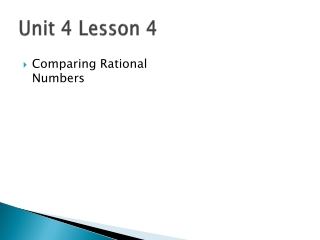DownloadDownload PresentationUnit 4 Lesson 4

# Unit 4 Lesson 4

Télécharger la présentation## Unit 4 Lesson 4

- - - - - - - - - - - - - - - - - - - - - - - - - - - E N D - - - - - - - - - - - - - - - - - - - - - - - - - - -
##### Presentation Transcript

1. Unit 4 Lesson 4 • Comparing Rational Numbers

2. Comparing Rational Numbers” • A RATIONAL NUMBER is a number that can be written as a fraction with an integer for its numerator and a nonzero integer for its denominator.

3. What Do You See? “EQUIVALENT FRACTIONS”

4. “Comparing Fractions” • Compare the fractions and . • When two fractions have different denominators, write equivalent fractions with common denominators. Then compare the numerators. = Same denominator, now compare numerators Therefore, 4/5 is greater 3/4. =

5. “Comparing Decimals” • To compare decimals, line up the decimal points and compare the digits from left to right until you find the place where the digits are different. Compare the fractions. Write < or >. Compare the fractions. Write < or >. < > 0.81 0.84 0.34 0.342 0.81 0.343 4 is greater than 1, so 0.84 is greater than 0.81 3 is greater than 2, so 0.34 is greater than 0.342 0.84 0.342

6. To write a decimal as a fraction, write the fraction as you would say the decimal Hundredths Ten-thousandths tenths 0.12345 Hundred-thousandths Thousandths

7. Say the decimal….Write it as a fraction • .5 • .65 • .22 • .2 • .225 Notice….when you change a decimal to a fraction the denominator is always 10, 100, 1000 (powers of ten!)

8. Order the Numbers from Least to Greatest 0.7011, 0.7, 0.71, 0.70, 0.7 Place numbers in order from least to greatest Line up numbers by the decimal point Write all decimals in the same place value 0.7011 0.7011 0.7011 0.7 0.7000 0.7 0.71 0.71 0.7100 0.70 0.7070 0.70 0.7 0.7777 0.7

9. What about fractions to decimals? You can write a fraction as a decimal by dividing the numerator by the denominator…..the fraction symbol means division!

10. Can be simplified to: .6 = .6 = Write .6 as a fraction BUT SO

11. To make a decimal…do the division! .75 .00 28 20 20 0

12. To make a decimal…do the division! .75 .00 28 20 20 0

13. Will be 1.4 1.2 will be Simplified to: Remember Whole numbers stay the same!

14. Using a Number Line Plot the numbers on a number line. Then order them from least to greatest. 0.2 0.4 0 0.5 0.625 0.67 1 1.5 1.5 In order them from least to greatest.

15. F D Using a Number Line Plot the numbers on a number line. Then order them from least to greatest. 0.3 0.21 0 0.5 0.6 0.6 0.85 1 1.5 In order them from least to greatest.

16. Comparing Rational Numbers 0 0.2 0.4 0.6 0.8 1 Course 2 Order the numbers from least to greatest. 4 5 , 0.93, and 0.9 The values on a number line increase as we move from left to right. 0.80 < 0.90 < 0.93 Place the decimals in order. 4 5 , 0.9, 0.93 0.93

17. Comparing Rational Numbers 0 0.2 0.4 0.6 0.8 1 Course 2 Order the numbers from least to greatest. 3 5 , 0.84, and 0.7 Write as decimals with the same number of places. 3 5 = 0.60 0.84 = 0.84 0.7 = 0.70 Graph the numbers on a number line. 0.84

18. Practice Page 138-139 odd, #11-39